Maths-
General
Easy

Question

# Write explicit formula.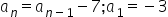Hint:

## The correct answer is: a_n=4-7n.

### Explanation: We have given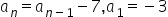We have to find the explicit formula of the given sequence. Step 1 of 1:We know that the recursive formula of an AP is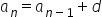, where d is common difference.Here we have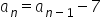So, d = -7Also, We have a1 = -3So, The explicit formula will be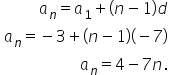.#### With Turito Foundation.#### Get an Expert Advice From Turito.